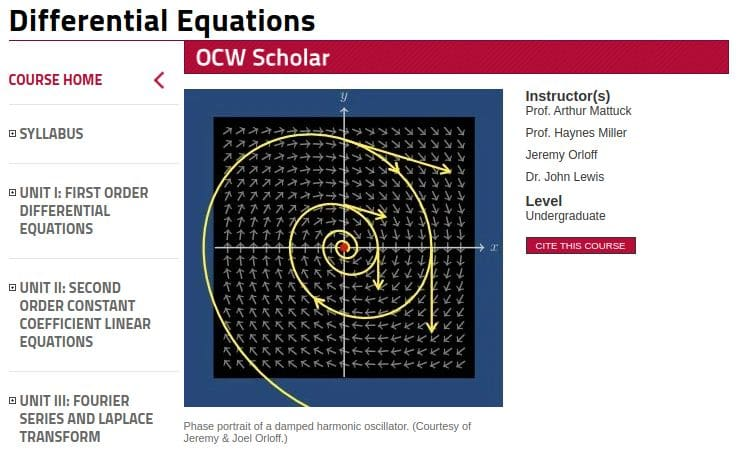# Free MIT Courses on Calculus: The Key to Understanding Deep Learning

Calculus is the key to fully understanding how neural networks function. Go beyond a surface understanding of this mathematics discipline with these free course materials from MIT.

Mathematics is undoubtedly the language of machine learning. Statistics is the very foundation upon which machine learning is built. Linear algebra is also a core contributor. However, in order to fully understand neural networks and deep learning, one must have some knowledge of calculus.

Like all things in life, there are varying levels of comprehension of calculus which could be deemed sufficient for differing depths of neural networks understanding. You could, for instance, feel justified in possessing a basic intuition of derivatives and an understanding of how backpropagation works for updating neuron weights based on these operations, should you simply use popular libraries to implement tried and true strategies for non-novel use cases. Translation: you don't a deep understanding of calculus in order to classify images using ResNet with Keras.

For an example of a simple but effective 30,000 foot explanation of what calculus is, here is the best single page introduction to calculus I have ever come across, a summary which would have saved me a lot of trouble a number of years ago:Calculus Made Easy 2nd ed, 1914, Chapter 1 (edited by article author to remove non-inclusive language).

It is difficult, perhaps, to link this to neural networks, but the basic intuition of calculus is achieved. If you are looking for a more full treatment of this branch of mathematics, you will want to seek out some more robust learning tools. Here are 3 courses and a textbook to help out, all from MIT's Open Courseware initiative, which will cover everything you need to know about calculus to understand deep learning — and far beyond.From the course's MIT Open Courseware page:

This calculus course covers differentiation and integration of functions of one variable, and concludes with a brief discussion of infinite series. Calculus is fundamental to many scientific disciplines including physics, engineering, and economics.

This course has everything you could hope for in terms of resources from an asynchronous online course:

• Lecture Videos with supporting written notes
• Recitation Videos of problem-solving tips
• Worked Examples with detailed solutions to sample problems
• Problem sets with solutions
• Exams with solutions
• Interactive Java Applets ("Mathlets") to reinforce key concepts

What's more is that this (and the other courses covered here) are OCW Scholar courses. What does that mean? OCW Scholar courses are noted for providing the full spectrum of resources needed for independent study, and boasts the full load of the same materials MIT students use in class.From the course's MIT Open Courseware page:

This course covers differential, integral and vector calculus for functions of more than one variable. These mathematical tools and methods are used extensively in the physical sciences, engineering, economics and computer graphics.

This is also an OCW Scholar course, and has the same full offering of course resource types as the previous course.From the course's MIT Open Courseware page:

The laws of nature are expressed as differential equations. Scientists and engineers must know how to model the world in terms of differential equations, and how to solve those equations and interpret the solutions. This course focuses on the equations and techniques most useful in science and engineering.

This, too, is an OCW Scholar course, and has the same complete set of materials as the previous courses.A full undergraduate level textbook on single variable and multivariable calculus, by Gilbert Strang of MIT. A good candidate to use as complement to the above courses, or a text-based study resource to use on its own.

1. Introduction to Calculus
2. Derivatives
3. Applications of the Derivative
4. The Chain Rule
5. Integrals
6. Exponentials and Logarithms
7. Techniques of Integration
8. Applications of the Integral
9. Polar Coordinates and Complex Numbers
10. Infinite Series
11. Vectors and Matrices
12. Motion Along a Curve
13. Partial Derivatives
14. Multiple Integrals
15. Vector Calculus
16. Mathematics after Calculus

A full PDF of the book can be accessed directly here.

A series of accompanying short videos titled Highlights of Calculus, demonstrating ways in which calculus is important in our lives, can be found here.

It's true that this is far more calculus than needed simply to understand deep learning, but once you start you might not want to stop.

Matthew Mayo (@mattmayo13) is a Data Scientist and the Editor-in-Chief of KDnuggets, the seminal online Data Science and Machine Learning resource. His interests lie in natural language processing, algorithm design and optimization, unsupervised learning, neural networks, and automated approaches to machine learning. Matthew holds a Master's degree in computer science and a graduate diploma in data mining. He can be reached at editor1 at kdnuggets[dot]com.

By subscribing you accept KDnuggets Privacy Policy

By subscribing you accept KDnuggets Privacy Policy

By subscribing you accept KDnuggets Privacy Policy